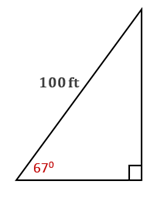Chapter 11.CT, Problem 11CTElementary Geometry For College St...

7th Edition
Alexander + 2 others
ISBN: 9781337614085

Solutions

Chapter
SectionElementary Geometry For College St...

7th Edition
Alexander + 2 others
ISBN: 9781337614085
Textbook Problem

A kite is flying at an angle of elevation of 670 with the ground. If 100 ft of string have been paid out to the kite, how far is the kite above the ground? Answer to the nearest foot.To determine

To find:

The height of the kite from the ground to the nearest foot.

Explanation

Given:

A kite is flying at an angle of elevation of 670 with the ground. If 100 ft of string have been paid out to the kite.

Approach:

In a right triangle, the sine ratio for an acute angle is the ratio oppsite sidehypotenuse.

Calculation:

From the diagram, hypotenuse = 100 ft, and the angle is 670.

Let the opposite side be x ft

Still sussing out bartleby?

Check out a sample textbook solution.

See a sample solution

The Solution to Your Study Problems

Bartleby provides explanations to thousands of textbook problems written by our experts, many with advanced degrees!

Get Started

Evaluate each expression: 83(92)217

Elementary Technical Mathematics

In Exercises 918, indicate whether the matrix is in row-reduced form. [101010001|346]

Finite Mathematics for the Managerial, Life, and Social Sciences

limx2x2(x1)1x = _____. a) 4 b) 1 c) 0 d) does not exist

Study Guide for Stewart's Single Variable Calculus: Early Transcendentals, 8th

The tangential component of acceleration for at t = 0 is: 0

Study Guide for Stewart's Multivariable Calculus, 8th